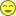### The Sabbath day

 Here is the mathematical proof the seventh day is the Sabbath day: (1 x 2 x 3 ) x ( 4 x 5 x 6 ) : ( 8 x 9 x 10 ) =  1  If you are not totally convinced, this one must eliminate any silly doubt: (1 + 2 + 3 + 4 + 5 + 6) + (8 + 9 + 10 + 11 + 12) = 21 + 27 = 48 = theseventhdaylack > and 72 minus theseventhdaylack =  1  Math is fun!